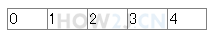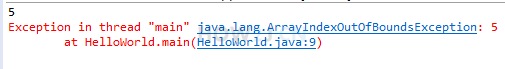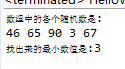8分35秒

## 声明数组

int[] a; 声明了一个数组变量。
[]表示该变量是一个数组
int 表示数组里的每一个元素都是一个整数
a 是变量名

public class HelloWorld { public static void main(String[] args) { // 声明一个数组 int[] a; } }
```public class HelloWorld {
public static void main(String[] args) {
// 声明一个数组
int[] a;
}
}
```

## 创建数组

new int

int c = 5; 这叫给c赋值为5

a = new int;public class HelloWorld { public static void main(String[] args) { //声明一个引用 int[] a; //创建一个长度是5的数组，并且使用引用a指向该数组 a = new int; int[] b = new int; //声明的同时，指向一个数组 } }
```public class HelloWorld {
public static void main(String[] args) {
//声明一个引用
int[] a;
//创建一个长度是5的数组，并且使用引用a指向该数组
a = new int;

int[] b = new int; //声明的同时，指向一个数组

}
}
```

## 访问数组public class HelloWorld { public static void main(String[] args) { int[] a; a = new int; a= 1; //下标0，代表数组里的第一个数 a= 2; a= 3; a= 4; a= 5; } }
```public class HelloWorld {
public static void main(String[] args) {
int[] a;
a = new int;

a= 1;  //下标0，代表数组里的第一个数
a= 2;
a= 3;
a= 4;
a= 5;
}
}
```

## 数组长度

.length属性用于访问一个数组的长度public class HelloWorld { public static void main(String[] args) { int[] a; a = new int; System.out.println(a.length); //打印数组的长度 a=100; //下标4，实质上是“第5个”，即最后一个 a=101; //下标5，实质上是“第6个”，超出范围 ,产生数组下标越界异常 } }
```public class HelloWorld {
public static void main(String[] args) {
int[] a;
a = new int;

System.out.println(a.length); //打印数组的长度

a=100; //下标4，实质上是“第5个”，即最后一个
a=101; //下标5，实质上是“第6个”，超出范围 ,产生数组下标越界异常

}
}
```

## 练习-数组最小值Or姿势不对,事倍功半! 点击查看做练习的正确姿势

0-100的 随机整数的获取办法有多种，下面是参考办法之一:

(int) (Math.random() * 100)

Math.random() 会得到一个0-1之间的随机浮点数，然后乘以100，并强转为整型即可。
public class HelloWorld { public static void main(String[] args) { int[] a = new int; a = (int) (Math.random() * 100); a = (int) (Math.random() * 100); a = (int) (Math.random() * 100); a = (int) (Math.random() * 100); a = (int) (Math.random() * 100); System.out.println("数组中的各个随机数是:"); for (int i = 0; i < a.length; i++) System.out.println(a[i]); System.out.println("本练习的目的是，找出最小的一个值: "); } }
```public class HelloWorld {
public static void main(String[] args) {
int[] a = new int;
a = (int) (Math.random() * 100);
a = (int) (Math.random() * 100);
a = (int) (Math.random() * 100);
a = (int) (Math.random() * 100);
a = (int) (Math.random() * 100);

System.out.println("数组中的各个随机数是:");
for (int i = 0; i < a.length; i++)
System.out.println(a[i]);

System.out.println("本练习的目的是，找出最小的一个值: ");
}
}
```

## 答案-数组最小值3分49秒 本视频采用html5方式播放，如无法正常播放，请将浏览器升级至最新版本，推荐火狐，chrome，360浏览器。 如果装有迅雷，播放视频呈现直接下载状态，请调整 迅雷系统设置-基本设置-启动-监视全部浏览器 (去掉这个选项)。 chrome 的 视频下载插件会影响播放，如 IDM 等，请关闭或者切换其他浏览器public class HelloWorld { public static void main(String[] args) { int[] a = new int; a = (int) (Math.random() * 100); a = (int) (Math.random() * 100); a = (int) (Math.random() * 100); a = (int) (Math.random() * 100); a = (int) (Math.random() * 100); System.out.println("数组中的各个随机数是:"); for (int i = 0; i < a.length; i++) System.out.print(a[i] + " "); System.out.println(); int min = 100; for (int i = 0; i < a.length; i++) { if( a[i] < min ) min = a[i]; } System.out.println("找出来的最小数值是:" +min); } }

HOW2J公众号，关注后实时获知最新的教程和优惠活动，谢谢。2020-10-26 答案
 小小小强_ 关于 JAVA 基础-数组-创建数组 的提问 答案 ``` int max = a; int min = a; System.out.println("数组中的各个随机数是:"); for (int j : a) { System.out.print(j + "\t"); } for (int j : a) { if (max < j) { max = j; } if (min > j) { min = j; } } System.out.println(); System.out.println("最大值："+max+" 最小值："+min);```

2020-10-14 答案
 lx213 正常运行 ```int a[]= new int; for (int i = 0; i < 5; i++) a[i]=(int)(Math.random() * 100); int min=a,j=0; for (int i = 0; i < 5 ; i++) { System.out.println(a[i]); if(a[i]< min) { min = a[i]; j=i; } } System.out.println("数组a中最小的元素是："+min+",它对应的下标是："+j);```

4 个答案

limerence

int y,x=100; int a[]=new int ; for(int i=0;i<a.length;i++) { a[i]=(int)(Math.random()*100); } for(int j=0;j<a.length;j++) { System.out.println("a"+"["+j+"]"+"="+a[j]+"、"); } for(int z=0;z<a.length;z++) { if(a[z]<x) { y=z; x=a[z]; } } System.out.println("最小数为："+x);

babab

public static void main(String[] args) { int[] nums = new int; for(int i=0; i<nums.length; i++){ nums[i] = (int) (Math.random() * 100); } for(int i=0; i<nums.length; i++){ if(i == nums.length-1) System.out.println(nums[i]); else System.out.print(nums[i]+","); } // 找数组的最小值, // 使用打擂台算法，先将第一个赋值给擂台变量，如果下一个比它小，那就取下一个的值 int min = nums; for(int i=1; i<nums.length; i++){ // 先循环遍历，然后依次比较每一个值 if(nums[i] < min) min = nums[i]; } System.out.println("数组中最小值为："+min); }

2020-09-17 第一题
2020-09-04 最小值
2020-08-26 成功了